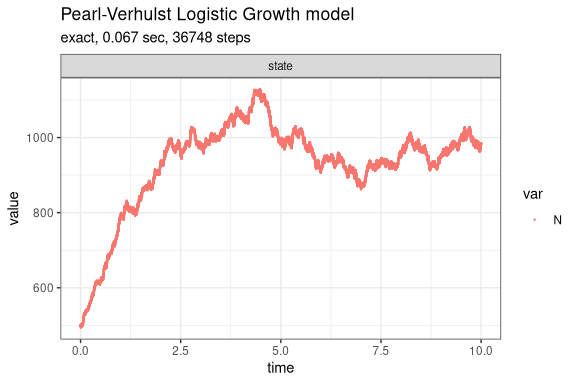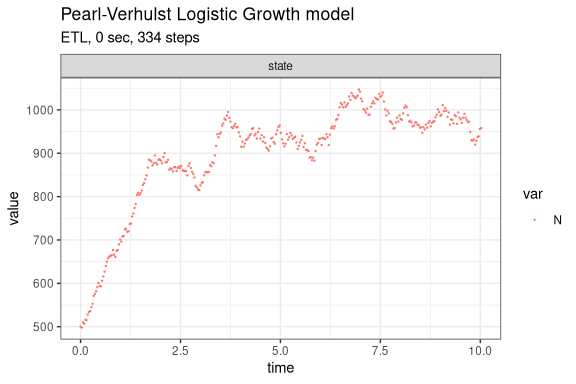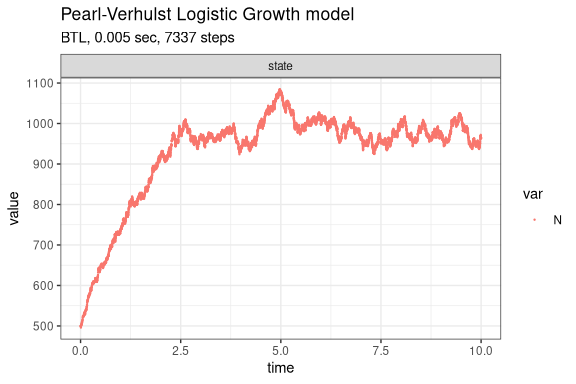# Pearl-Verhulst Logistic Growth model

The classical logistic-growth model (Kot 2001) assumes that the growth of a population decreases with increasing population size and is given by the following equation,

$\frac{dN}{dt} = rN \times \left(1 - \frac{N}{K}\right)$ where $$N$$ is the number (density) of indviduals at time $$t$$, $$K$$ is the carrying capacity of the population, $$r$$ is the intrinsic growth rate of the population.

This model consists of two reactions, birth and death, whose propensity functions are defined as:

• $$a_1(x) = bN$$
• $$a_2(x) = (d + (b - d) \times N / K) \times N$$

where $$b$$ is the per capita birth rate and $$d$$ is the per capita death rate.

Assuming $$b=2$$, $$d=1$$, $$K=1000$$ and $$X(0)=(500)$$, we can define the following parameters:

library(GillespieSSA2)
sim_name <- "Pearl-Verhulst Logistic Growth model"
params <- c(b = 2, d = 1, K = 1000)
final_time <- 10
initial_state <- c(N = 500)

The reactions (each consisting of a propensity function and a state change vector) can be defined as:

reactions <- list(
reaction("b * N", c(N = +1)),
reaction("(d + (b - d) * N / K) * N", c(N = -1))
)

Run simulations with the Exact method

set.seed(1)
out <- ssa(
initial_state = initial_state,
reactions = reactions,
params = params,
final_time = final_time,
method = ssa_exact(),
sim_name = sim_name
)
plot_ssa(out)Run simulations with the Explict tau-leap method

set.seed(1)
out <- ssa(
initial_state = initial_state,
reactions = reactions,
params = params,
final_time = final_time,
method = ssa_etl(tau = .03),
sim_name = sim_name
)
plot_ssa(out)Run simulations with the Binomial tau-leap method

set.seed(1)
out <- ssa(
initial_state = initial_state,
reactions = reactions,
params = params,
final_time = final_time,
method = ssa_btl(mean_firings = 5),
sim_name = sim_name
)
plot_ssa(out)